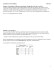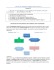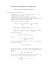# HYPOTHESIS TESTING

## Transcription

HYPOTHESIS TESTING
```HYPOTHESIS TESTING
Consider statements such as
 Teenagers aged 13-15 spend no more than 10 hours a week on Facebook
 The average weight of Australian men is the same as it was in 1990.
 Students from private schools have the same mean ATAR score as the Victorian average.
 The mean winter rainfall for the last 10 years is the same as the historical mean.
Our confidence about the probabilities of values drawn from normally distributed populations
and sampling distributions enables us to formally test hypotheses (or claims) such as these.
When we perform an ‘experiment’ we know there will be chance variation. For example, if we
toss a supposedly fair coin 100 times we would not be surprised to obtain 48 or 45 or perhaps
even 40 heads. However we would be surprised to obtain only 5 heads. If we were testing a
coin for ‘fairness’ we might even like to decide beforehand what we would consider a
reasonable number of heads. In hypothesis testing ‘reasonable’ is defined as what we could
expect 95% (or 99% or 90% etc) of the time.
In a hypothesis test we are concerned to assess how unusual our result is, whether it is
reasonable chance variation (obtaining 45 heads in 100 tosses of a coin) or whether the result is
too extreme to be considered chance variation (obtaining 5 heads in 100 tosses of a coin). The
experiment may consist of drawing a sample and comparing the sample mean with the
population mean for ‘reasonableness’. A hypothesis test is a formal process with the following
steps:
1.
State the null and alternative hypotheses
Ho:
̅ = μ [the sample mean is the same as the population mean allowing for chance variation]
Ha:
̅ ≠ μ [the sample mean is not the same as the population mean after allowing for chance
variation]
2.
A significance level α is chosen [α = 0.05  we are defining reasonable as what we can expect
3.
Tables or a calculator or a computer are used to find the
0.025
z-value that corresponds to the chosen significance level eg:
These are the thresholds for ‘reasonableness’ and are called
the critical values.
-1.96
4.
95% of the time]
0.025
0.95
1.96
The test statistic is the standardised difference between the sample mean (calculated
from the given data) and the known population mean: z =
̅
̅
[for a large sample where
σ is unknown]
5.
A decision is made regarding the ‘reasonableness’ of the test statistic:
Yes  Reject Ho
“Is the test statistic more extreme than the critical value?”
No  Do not reject Ho
6.
State your conclusion: There is (if you reject)/is not (if you do not reject) evidence to suggest
that….
NB: (i) The steps for hypothesis testing may differ from course to course so check with your
Program.
(ii) The decision relates only to rejecting or not rejecting Ho. Ha is not mentioned in the
decision, and we do not accept Ho or Ha.
Example
Because students had previously found a statistics course very difficult the average score
over many years was 48% with a standard deviation of 12%. A bridging program was
introduced and the 120 students that attended achieved a mean score of 50% in the final
exam. Is there evidence that the scores of those who attended the bridging program have
changed at a 99% level of significance?
1. Hypotheses: Ho: μ = 48
Ha: μ ≠ 48
2. α = 0.01 [level of significance 99% = 0.99]
3. Critical values: α = 0.01  z = -2.58 or z = 2.58
4. Test statistic: z =
̅
̅
=
= 1.83
√
5. Decision: Is 1.83 more extreme than 2.58? No, therefore do not reject Ho.
6. Conclusion: There is not evidence to suggest that the scores of those who attended the
bridging program have changed*. It is reasonable that the apparent improvement is due
to chance variation.
*[use the wording of the question]
Exercise
1. Repeat the example to decide if there is evidence at the 90% level of significance that
attending the bridging program is associated with the change in scores.
2. A random sample of 36 soft drinks from vending machines had an average content of
370ml with a standard deviation of 20ml. Test the null hypothesis that μ = 375 ml
against the alternative hypothesis μ ≠ 375 ml at the 99% significance level.
3. A bank manager has historical data that shows over lunchtime Mon –Fri the mean
number of customers that come into the bank is 32. Accordingly he believes he has no
need to change the number of tellers. However a branch survey conducted every
lunchtime over eight weeks found that the mean number of customers was 36 with a
standard deviation of 8.2. Conduct a hypothesis test with a 95% level of significance to
test whether the mean number of lunchtime customers has changed. What
recommendation would you make to the bank manager?
4. The manufacturer of ‘longlast’ batteries claims the mean lifetime of his batteries is 450
hours. A consumer interest magazine samples 100 batteries and finds that they have a
mean of 444 hours with a standard deviation of 28 hours. Do the sample data contradict
the manufacturer’s claim? [use α = 0.02]
Your answers should be set out and contain all the steps shown above. A brief outline of the main features is given
below
1.
2.
3.
Test statistic = 1.83  reject Ho: evidence of change in scores.
Test statistic = -1.5  do not reject Ho: difference consistent with chance variation
Test statistic = 3.09  reject Ho: evidence of increase in number of lunchtime customers and therefore
need more tellers
Test statistic = 2.14  do not reject Ho: the difference is consistent with chance variation and there is no
evidence to contradict the claim that the mean battery life is 450 hours.
```

### File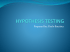### Document 6537334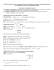### Population vs. Sample - Ms. Tomas` Math Page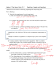### chapter 9 practice - faculty.piercecollege.edu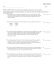### Chapter 11 and 12 Review 2015 ANSWERS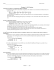### What is Hypothesis Testing? AP Statistics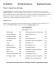### Inferential Statistics t and ScWk 242 – Session 9 Slides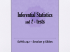### One-Sample Hypothesis Tests Review Problems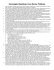### Math 116 - Final Review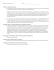### Chapter 6: Confidence Intervals and Hypothesis Testing Section 6.1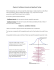### Chapter 7 Lecture Notes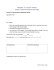### Sections 8-1 and 8-2 - Gordon State College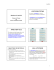### Final Review. More Problems. STAT 145 Problem 1. (formulation is